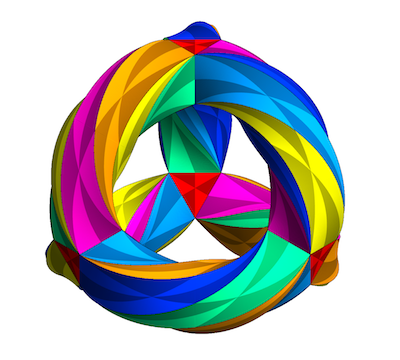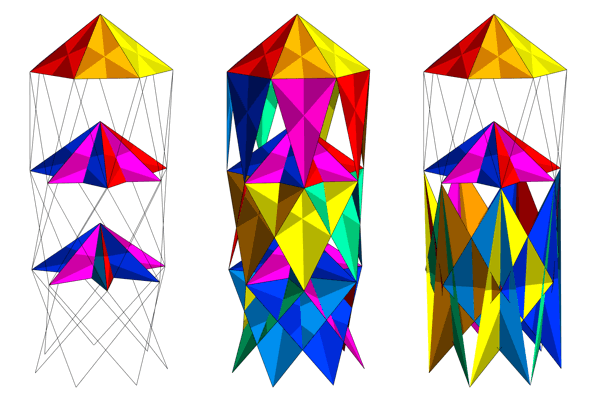# Klein’s Quartic Curve

## by Greg EganThis web page shows some illustrations I’ve made of a fascinating mathematical object known as Klein’s quartic curve. A more thorough explanation of the mathematics can be found at John Baez’s Klein’s Quartic Curve page and the references therein.

Klein’s quartic curve is a surface of genus 3, which is to say that it is like a 3-holed torus. As well as having that topology, the surface has a metric (a definition of distances and angles) of constant negative curvature, which means it has the local geometry of the hyperbolic plane. By drawing a 14-sided polygon in the hyperbolic plane and indicating that certain edges need to be connected to each other, you can specify the geometry of Klein’s quartic curve completely. There are a variety of other ways to describe the curve, most of them involving algebra and/or complex analysis. I’ve written a simple account of the construction of the curve via the wonderful quartic equation for it, u3v+v3w+w3u=0, in this page on Klein’s quartic equation.

One interesting thing about Klein’s quartic curve is that it can be tiled with a set of 24 identical regular heptagons, or alternatively with a set of 56 equilateral triangles. These two tilings are dual to each other, in the sense that the vertices of one tiling correspond to the faces of the other. In the heptagonal tiling, there are 24 heptagons, 84 edges and 56 vertices. In the triangular tiling, there are 56 triangles, 84 edges and 24 vertices. Note that these tilings give a Euler characteristic for the surface of χ = FE+V = 24–84+56 = –4, as required of a surface of genus 3 (the Euler characteristic for a closed, orientable surface of genus g is always χ = 2(1–g)).

These tilings make the surface into a Platonic surface, similar to the Platonic solids familiar from ordinary Euclidean geometry. A Platonic surface is tiled with identical regular polygons, with exactly the same number meeting at every vertex. In the heptagonal tiling, 3 heptagons meet at each vertex. In the triangular tiling, 7 triangles meet at each vertex.

The symmetry group (excluding reflections) of Klein’s quartic curve consists of the 168 symmetries of either of these tilings. In contrast to a sphere or an ordinary torus, which have continuous symmetry groups (e.g. you can rotate a sphere by an angle equal to any real number, around any of an infinite number of axes), Klein’s quartic curve has only these discrete symmetries. If you think about it, it makes sense that a surface of genus greater than 1 will only have discrete symmetries, because the presence of the extra holes will jam any kind of continuous sliding motion.

The image at the top of this page shows Klein’s quartic curve as a 14-sided polygon in the hyperbolic plane, divided into 56 triangles (some of which are split across the boundary of the 14-gon) and each of which in turn is divided into six smaller triangles of alternating dark and light shades. The 168 non-reflection symmetries allow us to move any given one of the 168 lighter triangles into any other such triangle, and similarly for the darker ones; if we include reflection symmetries, any given one of the 336 small triangles, of whatever shade, can be moved to any of the others.

The 24 heptagons fall into 8 sets of 3, such that rotations of one heptagon in the set are rotations of all 3 (but not by the same angle). The 56 triangles fall into 28 sets of 2, such that rotations of one are rotations of the other. And the 84 edges fall into 21 sets of 4, such that a rotation of 90 or 270 degrees exchanges two pairs of edges within the set, leaving none fixed, while a rotation of 180 degrees rotates each edge in the set. So the 168 elements of the symmetry group can be enumerated as follows:

• The identity element (no rotation).
• Rotations by between 1/7 and 6/7 turns of any of 8 different heptagons, giving 6 × 8 = 48 elements of order 7.
• Rotations by 1/3 or 2/3 turns of any of 28 different triangles, giving 2 × 28 = 56 elements of order 3.
• Rotations by 1/4 or 3/4 that exchange pairs of edges in any of 21 different sets, plus rotations by 1/2 that rotate the edges in any of these sets, giving 2 × 21 = 42 elements of order 4, and 21 elements of order 2.

Below is an image of the triangular tiling of Klein’s quartic curve embedded in ordinary 3-dimensional Euclidean space. In this embedding, the triangles are not all identical; it’s impossible to achieve that while placing the surface in ordinary space. However, this embedding does share a number of the symmetries of the real surface.This embedding is made as follows: take 4 triangular prisms, each with 2 triangular faces and 3 square faces, and place them at the vertices of a large tetrahedron. Then take 6 square antiprisms: solids with 2 square faces, and 8 triangular faces which join every vertex of each square face to an edge of the opposite one. Bend and twist the antiprisms and glue their square faces to those of the triangular prisms. The result is a surface of genus 3, with 8 flat triangular faces from the 4 triangular prisms, plus 6 × 8 = 48 curved triangular faces from the antiprisms, making a total of 56 triangular faces. These faces meet in sets of 7 around 24 vertices.

This embedding has the 12 rotational symmetries of a tetrahedron: the identity element, 2 rotations (of order 3) around each of 4 vertices, and 1 rotation (of order 2) around each of 3 pairs of edges. In addition, there are 12 more symmetries that come from combining these with a symmetry of order 2 that turns the whole tetrahedron inside out:Together, these 24 symmetries form a group that is algebraically the same as the group of rotational symmetries of a cube. It’s possible to immerse Klein’s quartic curve in 3-dimensional Euclidean space in a manner that has these 24 cubic symmetries, but the result intersects itself, making the topology less clear than it is in the embedding shown above.The 56 triangular faces of the dual tiling of Klein’s quartic curve can be divided into 7 sets of 8, which have the same symmetries as the 8 corners of a truncated cube. In all the diagrams on this page, these 7 sets are drawn in 7 different colours. So there are 7 different 24-element subgroups of the full symmetry group which are isomorphic to the symmetries of a cube. There is also a second way of dividing up the 56 triangles in this fashion, which yields what are effectively mirror images of the first way; the resulting “anticubes” have the same symmetry group, and so bring the total of 24-element subgroups to 14.

Klein’s quartic curve also has symmetries of order 7. These correspond to rotations of heptagons (or rotations around the heptavalent vertices of the triangular tiling), and the heptagons or vertices come in sets of 3 that are rotated together by different amounts.

The picture below shows three views of an immersion of the triangular tiling that makes this order 7 symmetry clear. In each view, some of the triangular faces have been stripped away to make it easier to see what’s going on. There are three vertices fixed by the rotation, lined up along a vertical line, each with 7 faces arranged around them in a kind of heptagonal pyramid. To limit the amount of self-intersection, these diagrams have been drawn with the 7 vertices of the base of the top pyramid also being represented at the very bottom of the picture; in other words, these pictures need to be interpreted as if the bottom is identified with the top (as if we’ve sliced through a torus and straightened it out into a cylinder).The second and third pyramids have their faces wrapped around, so that their edges form star polygons. These arrangements mean that a 1/7 turn that carries each vertex in the base of the top pyramid to one that it is joined to by an edge carries each vertex in the base of the second pyramid to one that it is joined to by traversing four edges, and each vertex in the base of the third pyramid to one that it is joined to by traversing two edges. So in a sense, the bottom pyramid rotates “twice as fast” as the top one, and the middle pyramid “four times as fast”. If the apex of the top pyramid corresponds to the centre of the 14-gon in the hyperbolic plane, the apexes of the other two pyramids correspond to the alternating points a and b that are the cusps of the 14-gon.

In the first view, all faces are stripped away except those involved with the three pyramids. In the second view, faces are shown that connect any two of the pyramids. In the third view, faces are shown that connect all three of the pyramids. Although the top-bottom identification removes some clutter, it can also be slightly confusing when counting faces and edges which are not shown twice, so it takes some care to check that each vertex here really does have 7 faces and 7 edges adjoining it.Science Notes / Klein’s Quartic Curve / created Friday, 27 May 2005 / revised Monday, 5 June 2017